Partial Derivatives And Euler's Equation MCQ Level - 2

# Partial Derivatives And Euler's Equation MCQ Level - 2

Test Description

## 10 Questions MCQ Test Topic wise Tests for IIT JAM Physics | Partial Derivatives And Euler's Equation MCQ Level - 2

Partial Derivatives And Euler's Equation MCQ Level - 2 for Physics 2023 is part of Topic wise Tests for IIT JAM Physics preparation. The Partial Derivatives And Euler's Equation MCQ Level - 2 questions and answers have been prepared according to the Physics exam syllabus.The Partial Derivatives And Euler's Equation MCQ Level - 2 MCQs are made for Physics 2023 Exam. Find important definitions, questions, notes, meanings, examples, exercises, MCQs and online tests for Partial Derivatives And Euler's Equation MCQ Level - 2 below.
Solutions of Partial Derivatives And Euler's Equation MCQ Level - 2 questions in English are available as part of our Topic wise Tests for IIT JAM Physics for Physics & Partial Derivatives And Euler's Equation MCQ Level - 2 solutions in Hindi for Topic wise Tests for IIT JAM Physics course. Download more important topics, notes, lectures and mock test series for Physics Exam by signing up for free. Attempt Partial Derivatives And Euler's Equation MCQ Level - 2 | 10 questions in 45 minutes | Mock test for Physics preparation | Free important questions MCQ to study Topic wise Tests for IIT JAM Physics for Physics Exam | Download free PDF with solutions
 1 Crore+ students have signed up on EduRev. Have you?
Partial Derivatives And Euler's Equation MCQ Level - 2 - Question 1

### If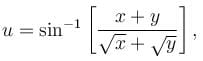then the value of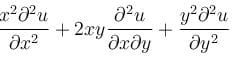is

Detailed Solution for Partial Derivatives And Euler's Equation MCQ Level - 2 - Question 1

u = sin-1(x + y/(√x + √y))

Put x = xt and y = yt

u = sin-1[(xt-yt)/(√xt + √yt)]

sinu = t1/2[(x+y)/(√x + √y)]

= t1/2 f(x,y)

This function sin u is homogeneous with degree 1/2.

By Euler's theorem

xux + yuy = G(u) = nf(u)/f,(u) = 1/2 tan u

xux + yuy = 1/2 tan u

x2uxx + 2xyuyy + y2uyy = G(u)[G'(u) - 1]

= 1/2 tan u [(sec2u - 2)/2]

= 1/4 tan u [(tan2u - 1)/1]

= 1/4 * sin u/cos u [(sin2u - cos2u)/cos2u]

x2uxx + 2xyuyy + y2uyy = -(sinu cos2u)/4ucos3u

Partial Derivatives And Euler's Equation MCQ Level - 2 - Question 2

###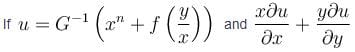then the value of is equal to

Detailed Solution for Partial Derivatives And Euler's Equation MCQ Level - 2 - Question 2

We have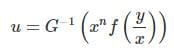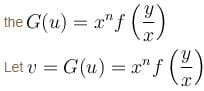v is homogeneous function of degree n then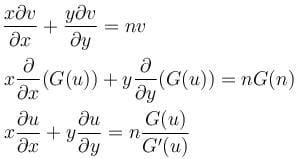The correct answer is: n(n - 1)

Partial Derivatives And Euler's Equation MCQ Level - 2 - Question 3

### The accompanying figure shows the graph of an unspecified function of f(x, y) and partial derivatives fx(x, y) and fy(x, y). Determine which is which and explain.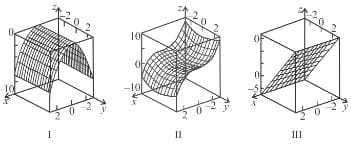Detailed Solution for Partial Derivatives And Euler's Equation MCQ Level - 2 - Question 3

The correct answer is: II - f(xy),  I - fx(x, y), III - fy(x, y)

Partial Derivatives And Euler's Equation MCQ Level - 2 - Question 4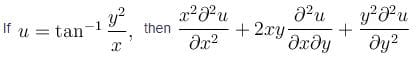Detailed Solution for Partial Derivatives And Euler's Equation MCQ Level - 2 - Question 4

We are given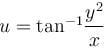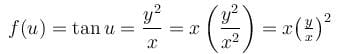f(u) is homogeneous function degree 1, then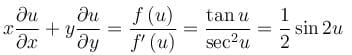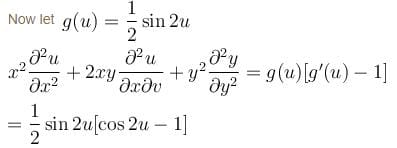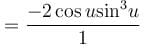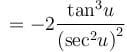[By dividing with cos3 u]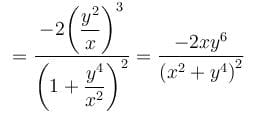The correct answer is: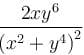Partial Derivatives And Euler's Equation MCQ Level - 2 - Question 5

If u = f(t) and v = f(t) and  t = φ (x, y)  then

Detailed Solution for Partial Derivatives And Euler's Equation MCQ Level - 2 - Question 5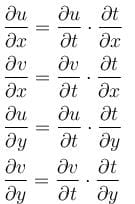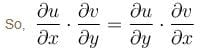The correct answer is: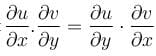Partial Derivatives And Euler's Equation MCQ Level - 2 - Question 6

If w = f(u, v) where u = x + y and v = x – y then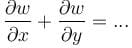Detailed Solution for Partial Derivatives And Euler's Equation MCQ Level - 2 - Question 6

We have w = f(u, v)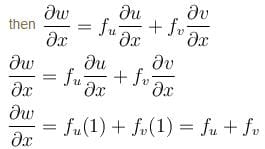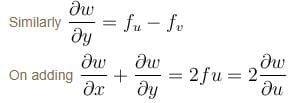The correct answer is: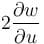Partial Derivatives And Euler's Equation MCQ Level - 2 - Question 7

If f is differentiable and z = u + f(u2v2), then

Detailed Solution for Partial Derivatives And Euler's Equation MCQ Level - 2 - Question 7

Let w = u2v2
then z = f(w) + u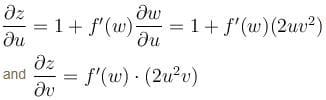So,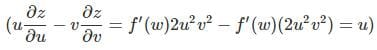The correct answer is: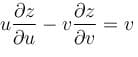Partial Derivatives And Euler's Equation MCQ Level - 2 - Question 8

If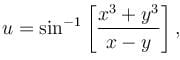then the value of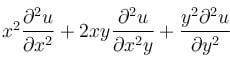is

Detailed Solution for Partial Derivatives And Euler's Equation MCQ Level - 2 - Question 8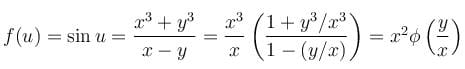f(u) is homogeneous function of degree 2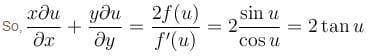Now, let g(u) = 2tan u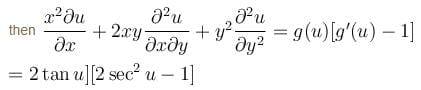The correct answer is: 2tan u(2sec2u –1)

Partial Derivatives And Euler's Equation MCQ Level - 2 - Question 9

If f(x, y, z) = 0 then the value of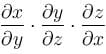equal to

Detailed Solution for Partial Derivatives And Euler's Equation MCQ Level - 2 - Question 9

(1) Differentiate with respect to y, I get:

0+F2+F3∂z/∂y=0

So

F3 ∂z/∂y = −F2

(2) Differentiate with respect to x, I get:

F1 + F2 ∂y/∂x + 0 = 0

So F2 ∂y/∂x = −F1

(3) Differentiate with respect to z, I get:

F1 ∂x/∂z +0 + F3 = 0

4) After some manipulations with the Fi, I get to the conclusion that

∂z/∂y∗∂y/∂x∗∂x/∂z=−1, so when evaluated with x, z, y respectively, conclusion is still true

Partial Derivatives And Euler's Equation MCQ Level - 2 - Question 10

Use the information the figure to find the first order partial derivatives of f at the point (1, 2)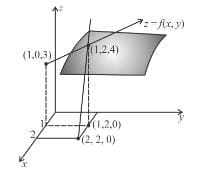Detailed Solution for Partial Derivatives And Euler's Equation MCQ Level - 2 - Question 10

The correct answer is: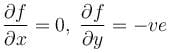## Topic wise Tests for IIT JAM Physics

217 tests
 Use Code STAYHOME200 and get INR 200 additional OFF Use Coupon Code
Information about Partial Derivatives And Euler's Equation MCQ Level - 2 Page
In this test you can find the Exam questions for Partial Derivatives And Euler's Equation MCQ Level - 2 solved & explained in the simplest way possible. Besides giving Questions and answers for Partial Derivatives And Euler's Equation MCQ Level - 2, EduRev gives you an ample number of Online tests for practice

217 tests